# Quantitative Aptitude Quiz for IBPS 2020 Mains Exams- 14th December

Directions (1-3): Study the given graph given below and answer the following questions
The graph given below shows the percentage of literates in three different villages in three
Years.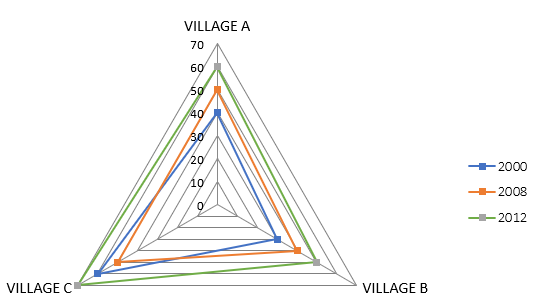Q1. If population of A in 2000, 2008 and 2012 is in ratio 2 : 3 : 4 and average of literate in 2008, 2012 and 2000 be 1410 then find population of village A in 2000.
(a) 1700
(b) 2000
(c) 2100
(d) 1800
(e) 2200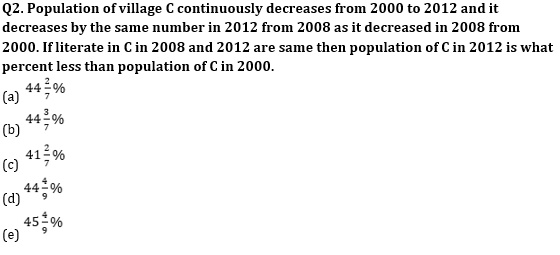Q3. Sum of literate from village B in 2000 and 2008 is 1530 and sum of literates in 2008 and 2012 is 2010 If sum of literates from villages B in all the given years is 2490 then find population of village B in 2008.
(a) 2625
(b) 2200
(c) 2000
(d) 2150
(e) 2050

Directions (4-6): Given below is the information about wind mills in four different villages A, B and C and D. Number of wind mills in villages A, B, C and D are 24, 20, 15 and 12 respectively. Number of electricity units produced in one week by one wind mill when they operate with maximum efficiency in village A, B, C and D is 2 lakh units/week, 80000 units/ week, 1 Lakh units/week and 1.5 Lakh units/week respectively. Number of houses in each village A, B, C and D are 540, 240, 150 and 350 respectively. Total units produced are consumed equally by each house in the village
→ Different number of winds mills are operate in four different weeks
In first week number of wind mills are operative in village A, B, C and D are 75%, 50%, 40% and 75% respectively. In second week it is 50%, 75%, 60% and 50% respectively. In third week it is 75%, 100%, 80% and 50% respectively. In fourth week it is 100%, 50%, 60% and 75% respectively.
→ Given below is the three ranges of efficiency of a wind mills (number of unit produced /Week by one mill)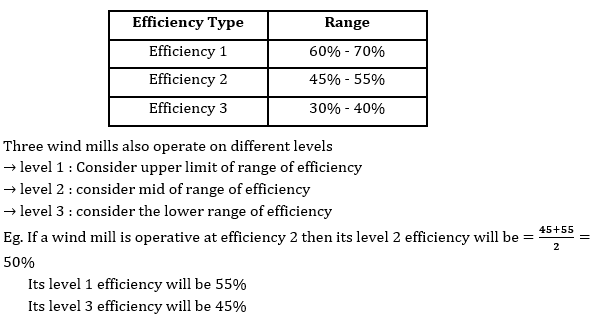Q4. What is the ratio of total production of village A in First week at level 1 of efficiency 2 to the total production of village B in second week at Level 2 of efficiency 1.
(a) 20 : 13
(b) 33 : 13
(c) 33 : 19
(d) 27 : 19
(e) 27 : 13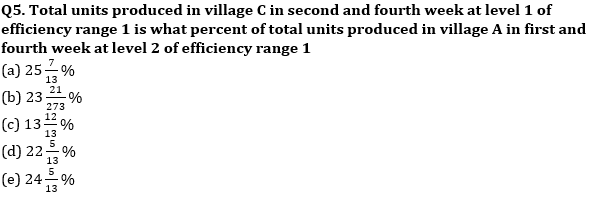Q6. What is the ratio of units consumed per house in village B in week 4 operating at level 3 of Efficiency range 3 to the units consumed per house in second week at level 1 of efficiency range 2 of the village C?
(a) 5 : 6
(b) 13 : 19
(c) 15 : 19
(d) 13 : 33
(e) 10 : 33

Directions (7-9): Line chart given below shows expense of five persons (in %) out of total income of two months. Income of persons is same in both months.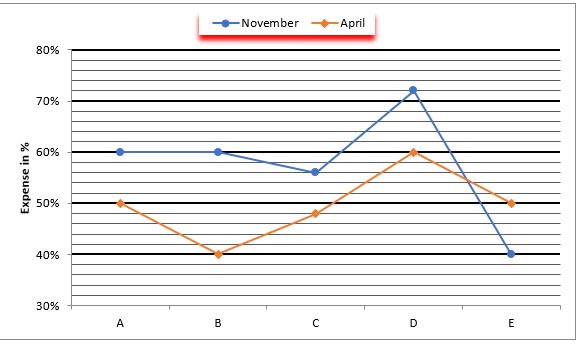Total Income = Expenditure + Saving

Q7. Find the difference between income of D and E ?
(I) Difference between expense of ‘D’ in November and saving of ‘E’ in April is Rs 3200.
(II) Difference between Saving of ‘D’ in April and Expense of ‘E’ in November is Rs 8000.

(a) Statement (I) alone is sufficient to answer the question but statement (II) alone is not sufficient to answer the questions.
(b) Statement (II) alone is sufficient to answer the question but statement (I) alone is not sufficient to answer the question.
(c) Both the statements taken together are necessary to answer the questions, but neither of the statements alone is sufficient to answer the question.
(d) Either statement (I) or statement (II) by itself is sufficient to answer the question.
(e) Statements (I) and (II) taken together are not sufficient to answer the question.

Q8. Average saving of ‘C’ in both months is Rs 19,200 while A’s income is 20% more than C’s income. Find expense of ‘A’ in the month of November
(a) Rs 9600
(b) Rs 19200
(c) Rs 38400
(d) Rs 24000
(e) Rs 28800

Q9. ‘B’ invested some amount of his saving in PPF account in November. Find the amount invested by ‘B’ in PPF account?
(I) Amount invested by ‘B’ in PPF is 62.5% less than amount expend by ‘B’ in April while difference between amount expend by ‘B’ in November and April is Rs. 16,000.
(II) ‘B’ invested 37.5% of his saving in PPF account while difference between saving of ‘B’ in November and April is Rs 16,000.
(a) Statement (I) alone is sufficient to answer the question but statement (II) alone is not sufficient to   answer the questions.
(b) Statement (II) alone is sufficient to answer the question but statement (I) alone is not sufficient to answer the question.
(c) Both the statements taken together are necessary to answer the questions, but neither of the statements alone is sufficient to answer the question.
(d) Either statement (I) or statement (II) by itself is sufficient to answer the question.
(e) Statements (I) and (II) taken together are not sufficient to answer the question.

Directions (10-13): Study the data given below and answer the following questions
Data is provided for 3 months for a water tank whose capacity is 600000 L to provide continuous water supply to a building. Water tank is first completely filled and then it gets completely emptied to supply water in a building. It supply water continuously to the building and is refilled again and again to provide continuous supply. In the building there are 40 flats in which all flats may or may not be completely occupied in the given three months.
November → Each flat is filled with a tap from which rate of flow of water is 250 L/h and only 50% flats are occupied in November. Water tank provides continuous water supply to these taps in whole month.
December → In this month 30 flats are occupied and tank gets emptied after 4 ⅙ days. Rate of flow from one tap in December is    A   % more or less than rate of flow from one tap in November.
January → Rate of flow of water from the taps is same as of November and gets emptied after supplying water to building for 100 hr. Number of flats occupied in January is    B   %

Q10. In November tank has to be filled how many times?
(a) 5
(b) 6
(c) 8
(d) 7
(e) 9

Q11. What is the value of A%
(a) 30%
(b) 25%
(c) 33 ⅓%
(d) 20%
(e) 15%

Q12. What is the value of B%
(a) 80%
(b) 40%
(c) 75%
(d) 60%
(e) 70%

Q13. In October efficiency of each tap decrease by 20% due to leakage as compared to efficiency of november and capacity of tank is reduced to 80%. In how many hours tank will be emptied in October if total occupied flats in October is equal to no. of occupied flats in December
(a) 65 hours
(b) 70 hours
(c) 30 hours
(d) 60 hours
(e) 80 hours

Directions (14-15): first bar graph shows total sales amount of three different companies in three different years and second bar graph shows the combined target sales amount of these 3 companies in these 3 years.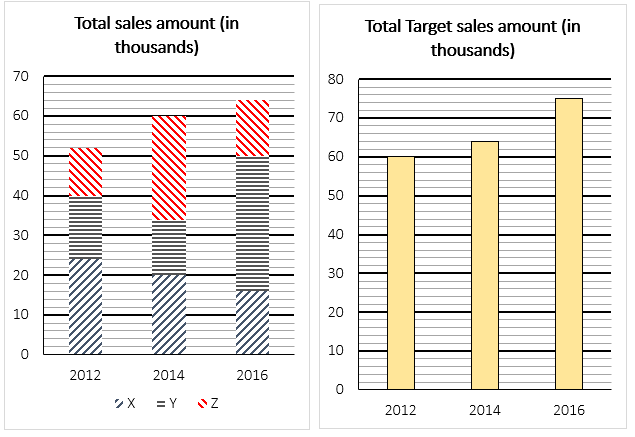Q14. If sales amount of company X and Y in 2012 increases by 20% and 25% respectively and sales amount of company Z remains constant, then find the difference between total target sales amount of all these companies in 2012 and sales amount of these 3 companies together as per the above condition? (in Rs.)
(a) 800
(b) 8000
(c) 8800
(d) 6000
(e) 3000

Q15. If the ratio of sales amount of company Y in 2016 to that of in 2018 is 17:15, then find the average of sale amount of company Y in 2014, 2016 and 2018?(in Rs.)
(a) 24000
(b) 25500
(c) 27000
(d) 26000
(e) 26500

Practice More Questions of Quantitative Aptitude for Competitive Exams:

###### Mains Quiz Study Plan for IBPS Exams 2020

Solutions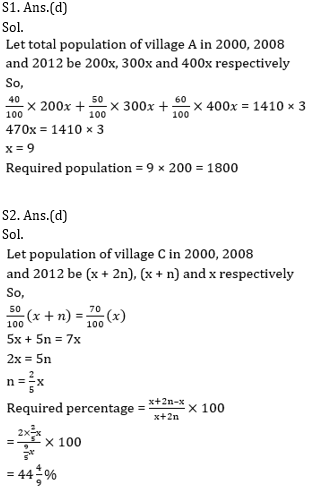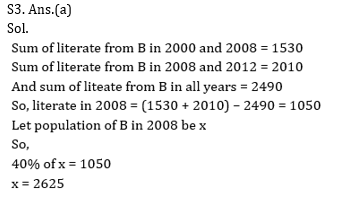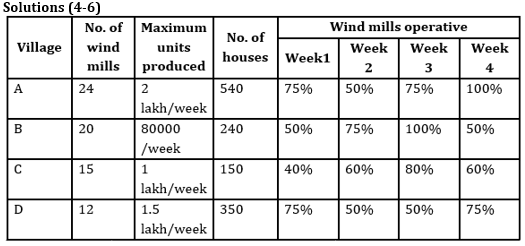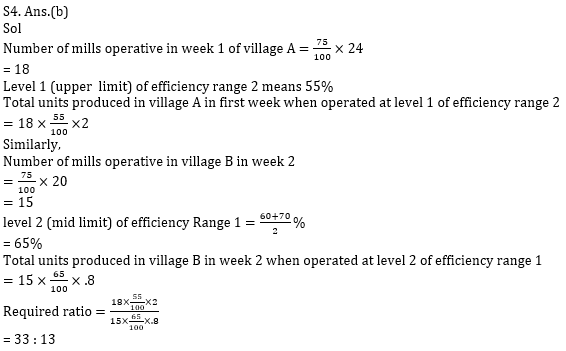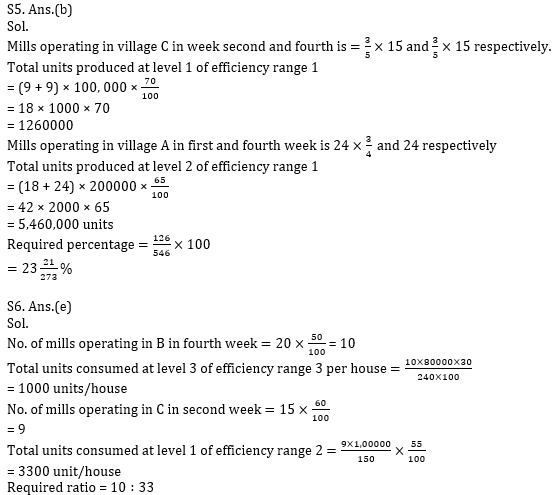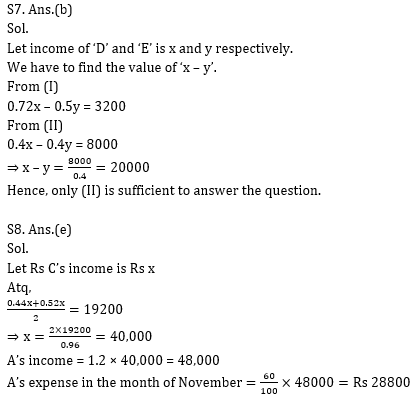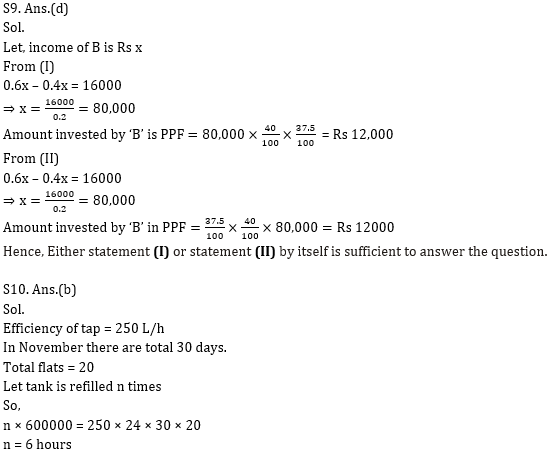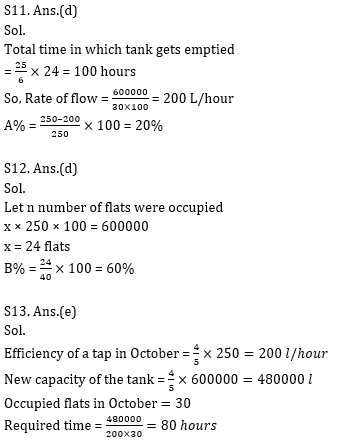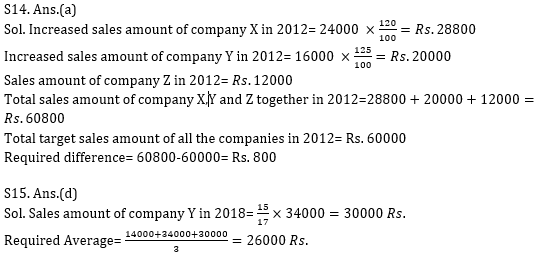Practice with Crash Course and Online Test Series for IBPS Clerk Prelims: